# RD Sharma Solutions for Class 11 Chapter 31 - Mathematical Reasoning Exercise 31.6

In this section, we will study the validity of statements. Checking the validity of a statement means checking when it is true and when it is not true. Students who aim to secure good marks in the board exams are advised to refer to RD Sharma Class 11 Maths Solutions. These solutions are readily available for easy access and download. RD Sharma books have been prescribed for years as a complete source of information to CBSE students, to develop their analytical and problem solving skills. They have proven to be essential for learning and developing the confidence that is required to face their exams. The RD Sharma Class 11 Maths Solutions explains the steps with precision, without missing out on essential aspects of solving a question. Students can download the pdf from the links given below.

## Download the pdf of RD Sharma Solutions for Class 11 Maths Exercise 31.6 Chapter 31 – Mathematical Reasoning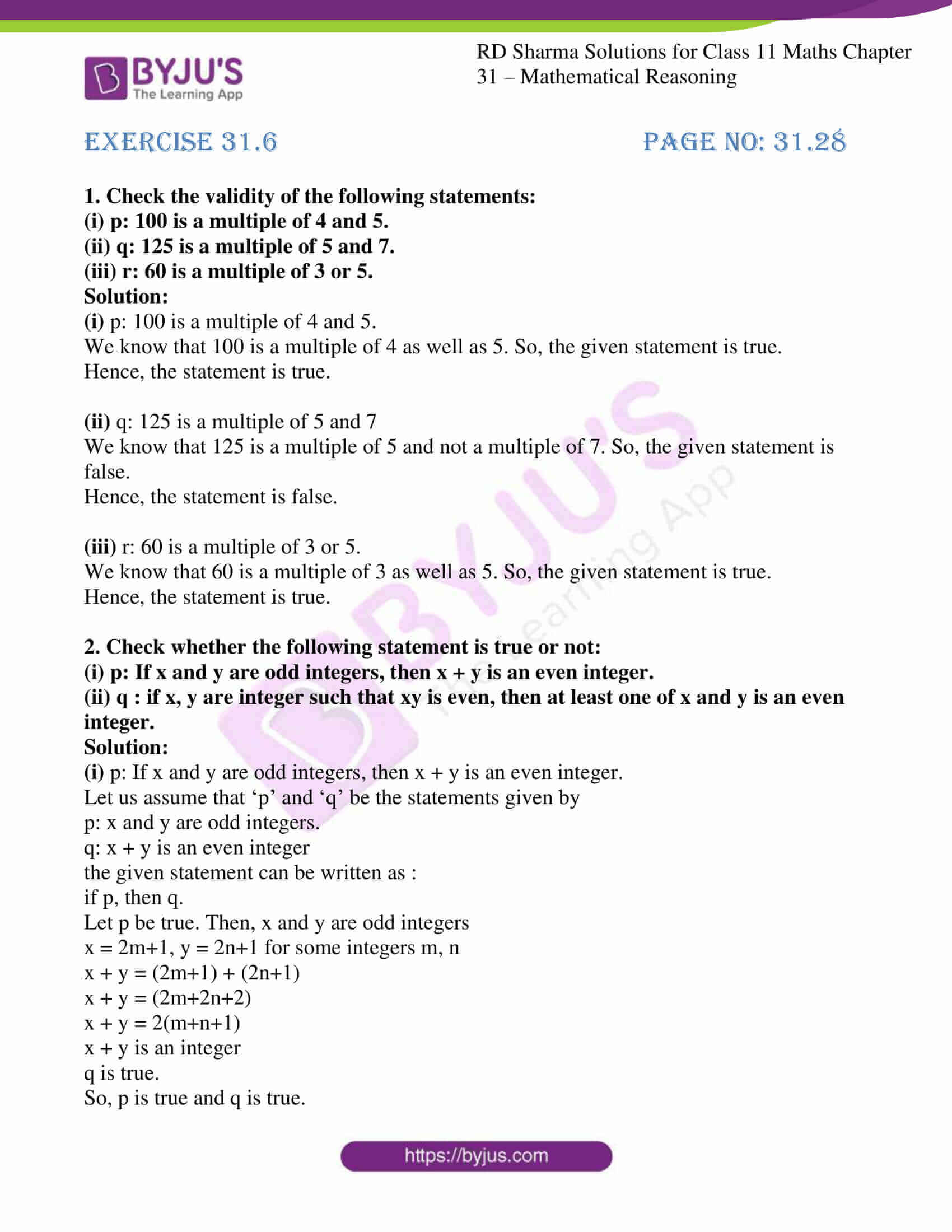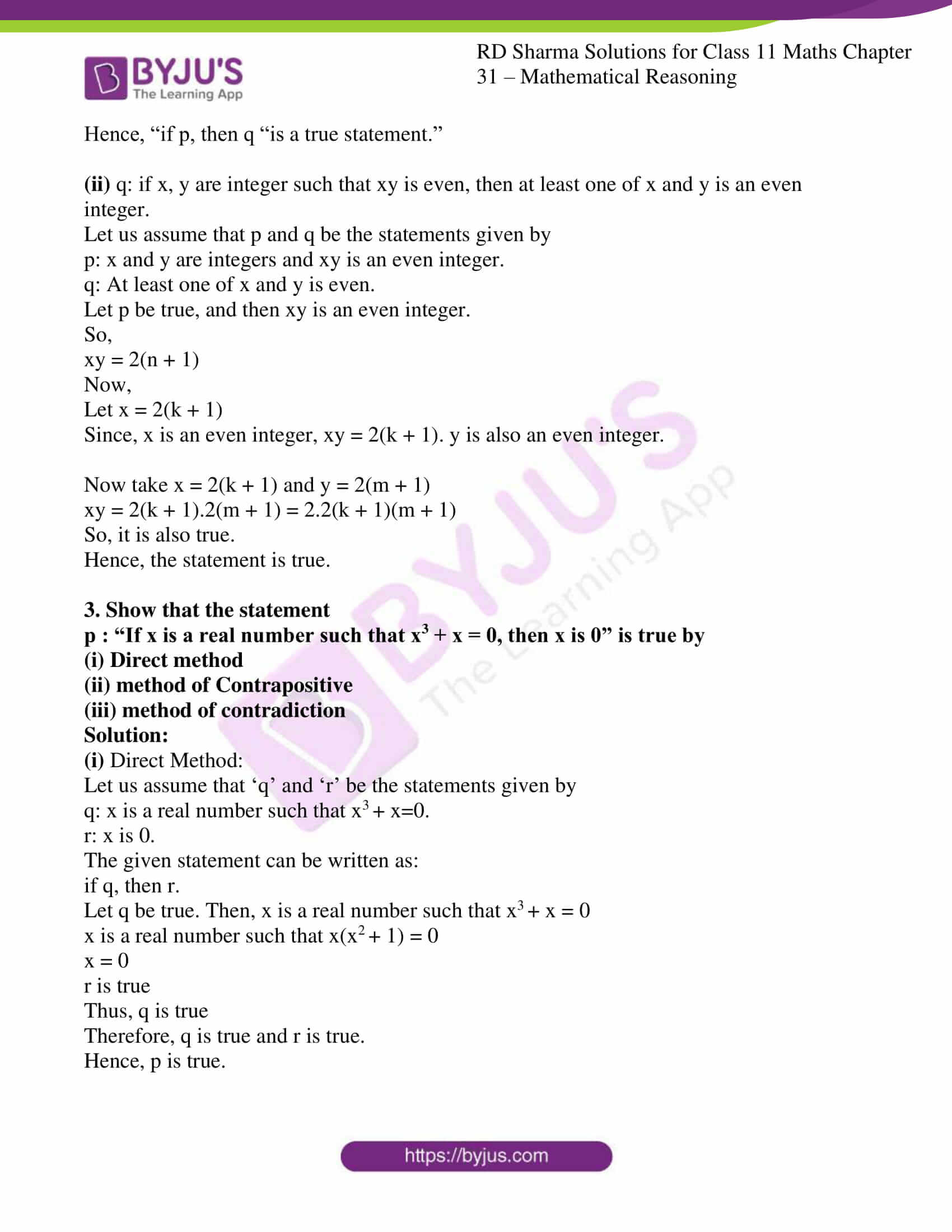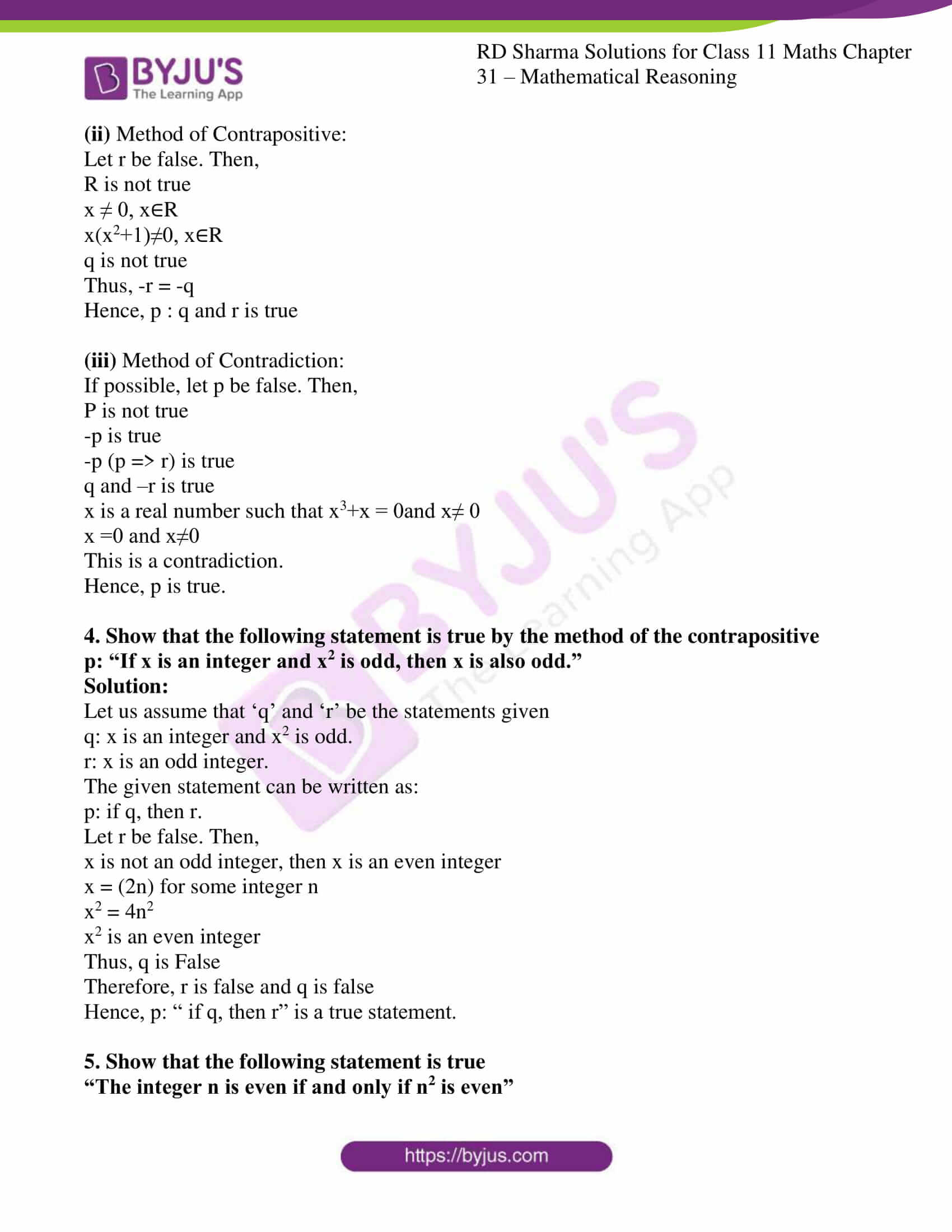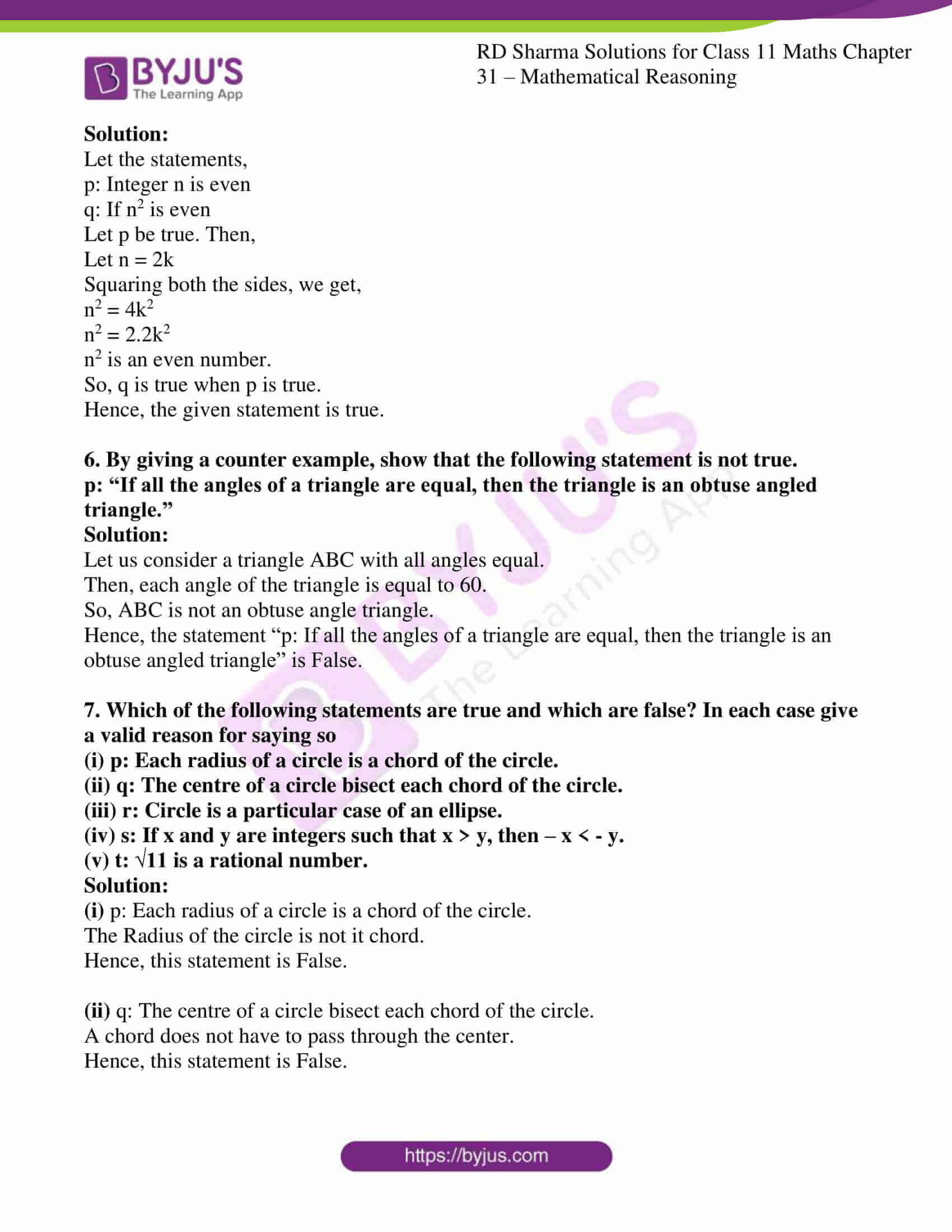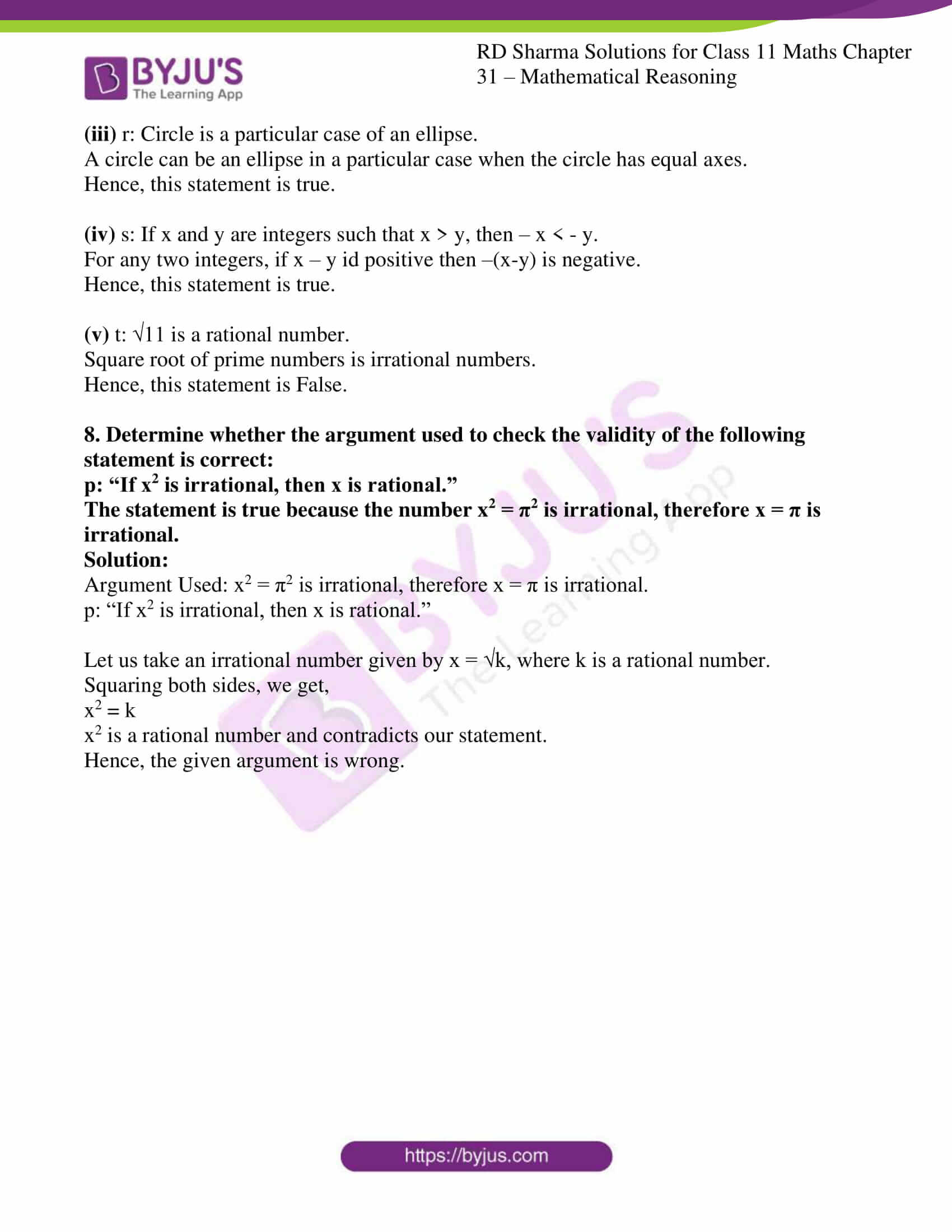### Access answers to RD Sharma Solutions for Class 11 Maths Exercise 31.6 Chapter 31 – Mathematical Reasoning

#### EXERCISE 31.6 PAGE NO: 31.28

1. Check the validity of the following statements:
(i) p: 100 is a multiple of 4 and 5.
(ii) q: 125 is a multiple of 5 and 7.
(iii) r: 60 is a multiple of 3 or 5.

Solution:

(i) p: 100 is a multiple of 4 and 5.

We know that 100 is a multiple of 4 as well as 5. So, the given statement is true.

Hence, the statement is true.

(ii) q: 125 is a multiple of 5 and 7

We know that 125 is a multiple of 5 and not a multiple of 7. So, the given statement is false.

Hence, the statement is false.

(iii) r: 60 is a multiple of 3 or 5.

We know that 60 is a multiple of 3 as well as 5. So, the given statement is true.

Hence, the statement is true.

2. Check whether the following statement is true or not:
(i) p: If x and y are odd integers, then x + y is an even integer.

(ii) q : if x, y are integer such that xy is even, then at least one of x and y is an even integer.

Solution:

(i) p: If x and y are odd integers, then x + y is an even integer.

Let us assume that ‘p’ and ‘q’ be the statements given by

p: x and y are odd integers.

q: x + y is an even integer

the given statement can be written as :

if p, then q.

Let p be true. Then, x and y are odd integers

x = 2m+1, y = 2n+1 for some integers m, n

x + y = (2m+1) + (2n+1)

x + y = (2m+2n+2)

x + y = 2(m+n+1)

x + y is an integer

q is true.

So, p is true and q is true.

Hence, “if p, then q “is a true statement.”

(ii) q: if x, y are integer such that xy is even, then at least one of x and y is an even integer.

Let us assume that p and q be the statements given by

p: x and y are integers and xy is an even integer.

q: At least one of x and y is even.

Let p be true, and then xy is an even integer.

So,

xy = 2(n + 1)

Now,

Let x = 2(k + 1)

Since, x is an even integer, xy = 2(k + 1). y is also an even integer.

Now take x = 2(k + 1) and y = 2(m + 1)

xy = 2(k + 1).2(m + 1) = 2.2(k + 1)(m + 1)

So, it is also true.

Hence, the statement is true.

3. Show that the statement
p : “If x is a real number such that x3 + x = 0, then x is 0” is true by
(i) Direct method
(ii) method of Contrapositive

Solution:

(i) Direct Method:

Let us assume that ‘q’ and ‘r’ be the statements given by

q: x is a real number such that x3 + x=0.

r: x is 0.

The given statement can be written as:

if q, then r.

Let q be true. Then, x is a real number such that x3 + x = 0

x is a real number such that x(x2 + 1) = 0

x = 0

r is true

Thus, q is true

Therefore, q is true and r is true.

Hence, p is true.

(ii) Method of Contrapositive:

Let r be false. Then,

R is not true

x ≠ 0, x∈R

x(x2+1)≠0, x∈R

q is not true

Thus, -r = -q

Hence, p : q and r is true

If possible, let p be false. Then,

P is not true

-p is true

-p (p => r) is true

q and –r is true

x is a real number such that x3+x = 0and x≠ 0

x =0 and x≠0

Hence, p is true.

4. Show that the following statement is true by the method of the contrapositive
p: “If x is an integer and x2 is odd, then x is also odd.”

Solution:

Let us assume that ‘q’ and ‘r’ be the statements given

q: x is an integer and x2 is odd.

r: x is an odd integer.

The given statement can be written as:

p: if q, then r.

Let r be false. Then,

x is not an odd integer, then x is an even integer

x = (2n) for some integer n

x2 = 4n2

x2 is an even integer

Thus, q is False

Therefore, r is false and q is false

Hence, p: “ if q, then r” is a true statement.

5. Show that the following statement is true
“The integer n is even if and only if n2 is even”

Solution:

Let the statements,

p: Integer n is even

q: If n2 is even

Let p be true. Then,

Let n = 2k

Squaring both the sides, we get,

n2 = 4k2

n2 = 2.2k2

n2 is an even number.

So, q is true when p is true.

Hence, the given statement is true.

6. By giving a counter example, show that the following statement is not true.
p: “If all the angles of a triangle are equal, then the triangle is an obtuse angled triangle.”

Solution:

Let us consider a triangle ABC with all angles equal.

Then, each angle of the triangle is equal to 60.

So, ABC is not an obtuse angle triangle.

Hence, the statement “p: If all the angles of a triangle are equal, then the triangle is an obtuse angled triangle” is False.

7. Which of the following statements are true and which are false? In each case give a valid reason for saying so
(i) p: Each radius of a circle is a chord of the circle.
(ii) q: The centre of a circle bisect each chord of the circle.
(iii) r: Circle is a particular case of an ellipse.
(iv) s: If x and y are integers such that x > y, then – x < – y.
(v) t: √11 is a rational number.

Solution:

(i) p: Each radius of a circle is a chord of the circle.

The Radius of the circle is not it chord.

Hence, this statement is False.

(ii) q: The centre of a circle bisect each chord of the circle.

A chord does not have to pass through the center.

Hence, this statement is False.

(iii) r: Circle is a particular case of an ellipse.

A circle can be an ellipse in a particular case when the circle has equal axes.

Hence, this statement is true.

(iv) s: If x and y are integers such that x > y, then – x < – y.

For any two integers, if x – y id positive then –(x-y) is negative.

Hence, this statement is true.

(v) t: √11 is a rational number.

Square root of prime numbers is irrational numbers.

Hence, this statement is False.

8. Determine whether the argument used to check the validity of the following statement is correct:
p: “If x2 is irrational, then x is rational.”
The statement is true because the number x2 = π2 is irrational, therefore x = π is irrational.

Solution:

Argument Used: x2 = π2 is irrational, therefore x = π is irrational.

p: “If x2 is irrational, then x is rational.”

Let us take an irrational number given by x = √k, where k is a rational number.

Squaring both sides, we get,

x2 = k

x2 is a rational number and contradicts our statement.

Hence, the given argument is wrong.